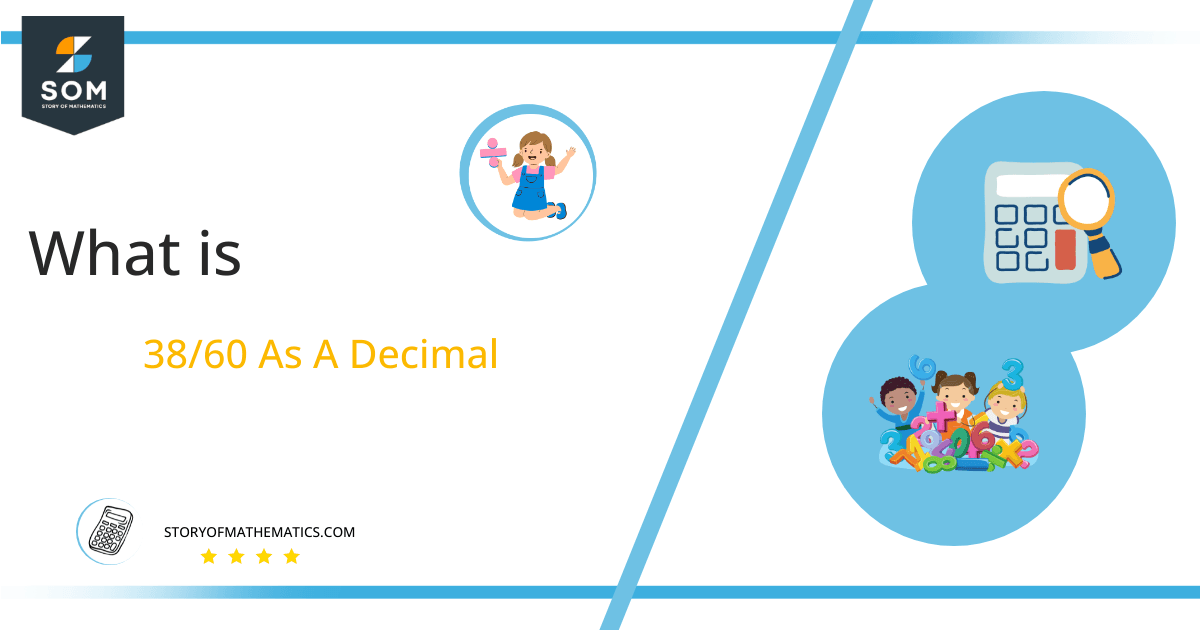# What Is 38/60 as a Decimal + Solution With Free Steps

The fraction 38/60 as a decimal is equal to 0.63333333.

Fraction is a mathematical expression used to divide an object or number into a certain number of equal pieces.  The numerator and denominator, which are two parts of the fraction, are divided to solve it and get the answer as a decimal value.Here, we are more interested in the division types that result in a Decimal value, as this can be expressed as a Fraction. We see fractions as a way of showing two numbers having the operation of Division between them that result in a value that lies between two Integers.

Now, we introduce the method used to solve said fraction to decimal conversion, called Long Division, which we will discuss in detail moving forward. So, let’s go through the Solution of fraction 38/60.

## Solution

First, we convert the fraction components, i.e., the numerator and the denominator, and transform them into the division constituents, i.e., the Dividend and the Divisor, respectively.

This can be done as follows:

Dividend = 38

Divisor = 60

Now, we introduce the most important quantity in our division process: the Quotient. The value represents the Solution to our division and can be expressed as having the following relationship with the Division constituents:

Quotient = Dividend $\div$ Divisor = 38 $\div$ 60

This is when we go through the Long Division solution to our problem.Figure 1

## 38/60 Long Division Method

We start solving a problem using the Long Division Method by first taking apart the division’s components and comparing them. As we have 38 and 60, we can see how 38 is Smaller than 60, and to solve this division, we require that 38 be Bigger than 60.

This is done by multiplying the dividend by 10 and checking whether it is bigger than the divisor or not. If so, we calculate the Multiple of the divisor closest to the dividend and subtract it from the Dividend. This produces the Remainder, which we then use as the dividend later.

Now, we begin solving for our dividend 38, which after getting multiplied by 10 becomes 380.

We take this 380 and divide it by 60; this can be done as follows:

380 $\div$ 60 $\approx$ 6

Where:

60 x 6 = 360

This will lead to the generation of a Remainder equal to 380 – 360 = 20. Now this means we have to repeat the process by Converting the 20 into 200 and solving for that:

200 $\div$ 60 $\approx$ 3

Where:

60 x 3 = 180

This, therefore, produces another Remainder which is equal to 200 – 180 = 20.

Finally, we have a Quotient generated after combining the three pieces of it as 0.63=z, with a Remainder equal to 20.Images/mathematical drawings are created with GeoGebra.